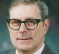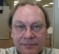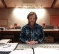## Calculating the Effective bit resolution from a .WAV recording?Started by 3 years ago16 replieslatest reply 3 years ago347 views

Good day,

I have various .WAV files of underwater recordings.  I used a ZOOM H1N recorder and a Hydrophone  (https://www.aquarianaudio.com/) to make the recordings.

I have managed to record some common dolphin, common bottlenose dolphin and Humpback whale sounds - which I can share if anyone is interested.

My main question is the following.  The H1N can save the file as a 24 bit WAV file.  However, I believe that this is an overkill.  Is there a way that I can theoretically "estimate"  the effective bit resolution?

(There are various noise sources in the .WAV file.  The main noise source is "shrimp snapping".  The other is wind noise, that is generated by the way we deploy - our ethics clearance only allows dipping hydrophones and floating buoys ...)

I thought of taking the 24 bit file and converting it to 16 bits using the Linux SOX command.  I can then read the two files into MATLAB/Python, and subtract the two signals from each other.  I can then calculate the power of this signal.  Would I be able to learn anything from this?

(I assume I would be able to perform the 24 bit to 16 bit conversion in Matlab as well ...)

Any thoughts and suggestions will be greatly appreciated,

Jaco

[ - ]I assume that the data originally came from an A/D converter with some number of bits. If no further processing was done after the recording, such as filtering, you should be able to compute a histogram of the levels recorded and therefore see the smallest difference and the largest value.

[ - ]The input stages of these devices usually have a dynamic range (difference of loudest possible input signal to noise floor) of less than 120dB. That's about 20bits of resolution. So I'd say you could safely through away the lowest 4bits, probably more. If normalizing your signal doesn't harm, you can first normalize it and then trhow away the lowest (4 + log2(normalization_gain)) bits away.

[ - ]Thanks for this.

Is there a way that I can empirically verify the "quality" of the signal after reducing the bit resolution?

Say x_24 is the samples at 24 bit resolution and x_16 is the samples at 16 bit resolution.  Would the power of x_diff = x_24 - x_16 tell me anything?  If this is lower than the quantization noise, I can then still drop my bit resolution?

[ - ]dudelsound has the right concept. Keep in mind that every bit of your signal is 6dB, and your device will have a dynamic range that defines how many bits are actually used. So, if your recording device has a 96dB dynamic range, it will only use 16 bits of the 24 bit signal. Now you have to determine where those 16 bits are. Unused bits in the MSB are unused headroom, and unused bits in the LSB are the noise floor. I'd start by just taking the absolute value of the signal and looking for bits in the MSB and LSB that never change. If bit 22 is always zero, you have 6dB of headroom you never used, and can toss it. If bits 0-4 are always one, you've found your noise floor. Everything in between is signal or signal+other noise. If the signal is riding on DC jitter or hum you'll have to filter that out first. A straight 24-to-16 bit conversion usually just tosses the 8 LSB bits, and there could be signal there. You need to determine the dynamic range of your signal and the recording level of your ADC first.

[ - ]Thanks,

So I did the following experiment on one of the files:

*** Begin Matlab code ***

%Matlab code

clear
clc

yy = y(:,1)*2^23;  %Shifting the data left

yy_i = find(yy > 0);   %Discard 0 and negative values
yy = yy(yy_i);

yy_32 = cast(yy,'uint32');  %Cast to 32 bits (Assume 24)

buf = [];
for i = 1:32

%i

b = bitget(yy_32,i);
b_n = sum(b);

buf = [buf; b_n];

end

buf

*** End Matlab code ***

I was not sure how Matlab would handle the 'single'/float cast to uint32, so I only worked with values greater that 0.

Here is the output of the variable buf.  buf(1) -> bit1, ... buf(32) -> bit 32

buf =

20512787
20516816
20517164
20517959
20506856
20507810
20499701
20484619
20457899
20396257
20275696
20045760
19567797
18605482
16574295
12110458
6669019
3180573
1298884
480207
100616
7162
3335
0
0
0
0
0
0
0
0
0

There was a total of 41028845 candidate samples  (samples greater than 0).  Thus, almost half (50%) of the samples have the lowest bit equal to 1, while the rest of the samples have the lowest bit equal to 0.  This is almost true up to bit 5 ...

So, at a first glance it seems as if the setup has a bit resolution of 23 bits.  The signal's amplitude was small for most of the time, so I didn't expect a lot of activity in the higher bits.

[ - ]Unfortunately I'm not a Matlab user so I can't check your code. However, you might want to toss the negative values first before shifting. Your results are exactly what I'd expect to see for non-DC-shifted sinusoidal twos-complement signed values, regardless of signal level. Unused lower bits won't change, and unused higher bits will toggle at roughly half the total number of samples. If the results come out nearly the same, as dudelsound recommended your signal may already be normalized to full-scale, and indeed you can just toss the lower bits. It appears to me most of your signal is in the upper 16 bits, and below that is your noise floor. Doing as you suggested in your original post (done correctly) will allow you to extract out whatever is in the noise floor.

[ - ]Seriously, download and install Audacity. It's a great tool for exploring and playing with audio and literally "shows" you graphically the signal. I went to the microphone website you mentioned and it had a humpback whale recording and as I imagined, it a "good old zero mean signal" (i.e. the positive peaks are about equal to the negative peaks with "zero" about halfway between) and a portion of it looks like this:This tool will let you scale the signal in amplitude and time. I think that everything you want to do (other than the unspecified "filtering") can be done in Audacity.

[ - ]I am audio person not math/DSP. But generally in audio one wants to keep the highest sample and bit rate possible at all times. If you have these 'ambient' noise you trying to limit or remove. Things like that are attempted in audio software, we would use a processor to sample the shrimp sound, and then that processor plugin, will use math, to attempt to remove those sounds. This can be done in degrees, more or lesser. To find the balance that does not alter the sounds you wish to keep. It can very successful or it can be not acceptable, depends on the nature of the ambient sound itself, how similar it is to the sounds you want to hear. And generally we want to do this at the highest resolution possible - 96/24 is perfect for that process. If that is the direction you go in. Smaller files can be made later. Bur the original and precoessing should be done at highest rates.

[ - ]On 16 bit VS 24 bit.

Suppose you divided a mile into 64K pieces.  Each piece would be 5280/65536 ~=~ 0.08 feet ~=~ 1 inch.

If you are measuring a changing length that is varying by several feet per sample, does dividing that inch into 256 pieces really make a difference in anything but the most precise of applications?

Your ambient noise level is probably much higher.

To capture the tones of the sounds more accurately, it is much better to increase the sampling rate.  Especially in this application if you are dealing with squeals and such which have high frequency components.

Don't store your files with a compressed format if you are going to do analysis on them.
[ - ]Thanks for all the replies.

To clarify, I believe the ZOOM H1N uses an A/D (actually an Audio Codec) that samples at 24 bit resolution.  I currently sample at a sampling rate of 96kHz.  (For whale sounds, my signals of interest is below 2 kHz, so I am still contemplating dropping the sampling rate a bit.  For dolphins, the whistles go quite high, up to 24 kHz for the two species I encountered.)

I am recording with .Wav format, as I do not want to perform any lossy compression.  (I stay away from MP3).

We use Hidden Markov Models to perform classification and detection.

I am actually interested in using a Microprocessor with an external A/D or perhaps an Audio Codec.  One of the questions I have is what bit-resolution would be adequate for our algorithms.

There are commercial systems that sample at 16 bits / sample.  There is an opensource hardware project  "Audiomoth", which used the Microprocessor's built in A/D and samples at 12 bits.

From my own recordings, it would be nice to see what the actual (effective) bit-resolution is for the hydrophones that I use.

Thanks,

Jaco

[ - ]To calculate the effective number of bits (ENOB) of a recording, scan all the samples to determine the min and max values. From that, then:

ENOB = Ceiling(log(max - min)/log(2))

This will be the power of 2 (i.e. number of bits) needed to capture that recording without loss.

[ - ]Hi artmez,

Actually, S/N in the ENOB formula is full-scale sine power relative to the total noise power.  So you have to integrate the noise power to find the S/N.  Note that the exact formula is

S/N = 1.76 + 6.02*ENOB   dB

The sine power relative to noise density (1 Hz) is:

S/No = -1.25 + 10log10(fs) + 6.01*ENOB    dB (1 Hz)

See, for example, Digital Signal Processing in Comm. Systmes by Frerking, p79.

regards,

Neil

[ - ]I've been caught! Thanks for that. I do forget that's the official way to do this. The 6.02 in dB is essentially the power of two squared.

What I showed is a way to simply determine how many bits are needed to encode a specific range without regard to SNR and works with the underlying number ranges only, right?

[ - ]I don't know.  I usually deal with signals that are ac-coupled.
[ - ]Zero mean or AC coupled -- they're the same. But for the range analysis, they needn't be zero mean. Composite signals and look really strange (e.g. white noise). If "white noise" is "stationary", then for infinite time, it is zero mean, but for a small time slice it most likely will not be zero mean. The same is true for even simple sinusoids. Take for example a sample of 1/4 of a cycle of any sine wave with arbitrary phase. Its DC value will vary widely but will never be zero (for an AC coupled signal).

Warning: the following will be extremely boring to most readers!

I used my scheme to analyze a nonlinear signal from an ILS (instrument landing system) field monitor subjected to various perturbations due to various aircraft and ground based traffic (plane and vehicles) that caused reflections, diffraction, etc. of the monitored signal used for critical CAT II and III landings, including zero visibility. I had over a thousand sets separate "events" of over 250,000 measurements total that were all deemed "normal" (i.e. shouldn't result in alarm shutdown) whereas about 25% of these did result in alarms that would affect runway operation. I had to analyze these for "something" that would let me "filter" (also nonlinear) these to automatically and temporarily bypass "known acceptable conditions" to allow continuous monitoring of that field monitor signal. This began by writing a graphical analysis program that allowed me to easily "zoom" in on signals in both time and amplitude domains. That, and a lot of "time" allowed me to come up with a solution that has be in operation since 1993 on thousand of airport runways around the world. It was hard, but it was so much fun too. One of the tools I used employed the mechanism I described to scale the signals for graphical analysis.

OK, that last part wasn't too applicable to the subject at hand, but it's where I first used the concept (that I can recollect).

[ - ]